Number Sense: The Power of Patterns

# Number Sense: The Power of Patterns

A child more readily learns material if it is embedded within a pattern. Brain research tells us that the human brain is a pattern-seeking organ, and we have only to watch children at work in pattern discovery to know that this is true. Patterns provide order for seemingly chaotic material and help us make sense out of seemingly random facts. Pattern discovery also provides the global framework so important to many children. The greatest benefit of pattern discovery is that it eliminates the need for memorization of facts. Through practice, students will come to remember the pattern visually, like having a mental snapshot stored in their minds, so they can locate individual facts from within that global picture.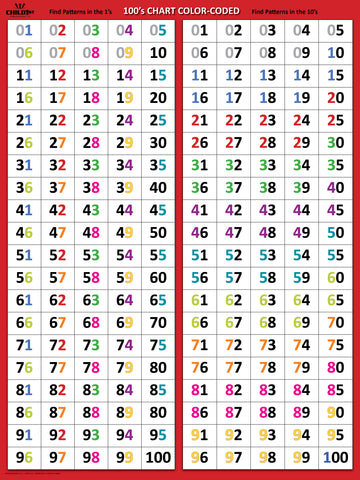Our 100s Chart Color-Coded poster is a great resource for helping students with number sense using patterns. The chart on the left is color-coded for pattern-seeking in the 1s columns, while the chart on the right is color-coded for pattern-seeking in the 10s columns. It is arrayed in rows of 5 to facilitate counting money and telling time. The following activities are sure to add fun and understanding of numbers for your students.

## Pattern-Seeking Activities

Start with the 1s chart, find patterns, and notice how the numbers are alike.In the first column, note the repeating numbers 1, 6, 1, 6, 1, 6, and so on.

“Do you think that maybe the 1 in the first row fell straight down and became curved at the bottom, forming the number 6?”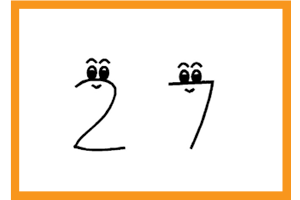In the second column, the pattern that repeats is 2, 7, 2, 7.
“Do you see that the two numbers contain a slant from top right to bottom left?”In the middle column, the pattern that repeats is 3, 8, 3, 8.

“How are the 3 and 8 alike?” (The 3 is like the 8 but with one side cut off!)In the 4th column, the repeating pattern is 4, 9, 4, 9.

“Look at the 4, 9 pattern. How do the numbers look similar?”
(Both numbers are top-heavy with a line extending down to complete the number).In the last column, the repeating pattern is 5, 0, 5, 0.
“In this column, both numbers have a round part.”

Go to the 10s chart and let's look for patterns.

• In the first four 10s columns, the numbers don’t alternate every other one. They alternate by twos. In these four columns, the repeating pattern is 0, 0, 1, 1, 2, 2, 3, 3, … all the way to 9, 9.

• In the 5th column, the pattern is the same except for how the pattern starts and ends. Here is the pattern: 0, 1, 1, 2, 2, 3, 3, 4, 4, 5, 5, 6, 6, 7, 7, 8, 8, 9, 9, 10.

Look for patterns between numbers on the whole chart.

• If you point to any number on the 100s chart and want to add 10, just skip down two places. Try it. Point to the 4 in the top row. 4 + 10 is the number two rows down: 14.• If you point to any number on the 100s chart and want to add 5, just look at the number just below the number you are pointing at. Try it. Point to 42. 42 + 5 is the number right under 42: 47. You will know it is 47 because the repeating pattern in the 10’s column is 4, 4, and the repeating pattern in the 1s column is 2, 7. So you have 42, 47.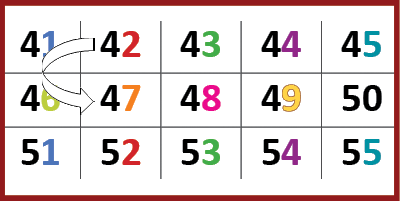• How would you find the answer to the problem 24 + 15? Point to the 24 and then skip down to the 3rd row under 24. So, 24 + 15 is 39.• You can play with all sorts of addition or subtraction problems using the 100s chart. For example, any number + 6 will be the number just below and 1 to the right. Try it. Put your finger on 17 and add 6 to it. The number just below 17 is 22, but the number to the right of 22 is 23. So, you basically added 5 and one more, which is 6.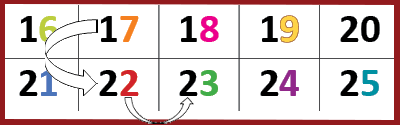• How would you move on the 100s chart if you are adding 7? (You would move down one, and then move 2 to the right).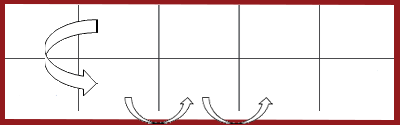• How would you use the 100s chart to subtract? Well, if you move down when you add, you can subtract simply by moving UP instead! Try it. If you point to 38 and want to subtract 5, just move UP one row and you will land on 33.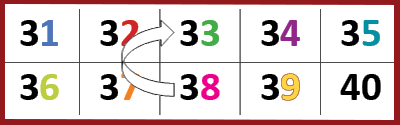• Notice that in the 5th column, you can say every number in the column – and you will be counting by 5’s! If you name every other number in that column, you will be counting by 10s. How would move if you want to count by 15s? By 20s? (For 15s you would skip two rows, and for 20s you would skip three rows).

## Other Applications

• Allow time for students to discover patterns during lessons.

• Model your own habit of pattern discovery in everything you teach students. Ask, “How can we arrange the problems so that they form a pattern?”

• Give your students groups of facts at the same time so they can discover relationships between the problems, rather than giving them isolated problems with no apparent connection to each other.• Teach groups of facts that have something in common. (i.e. Present problems that have the same sum, sums that increase by one, or an addend that is the same.)

• Study and practice math facts within a pattern first, then mix them up, and finally, combine them with other, previously learned facts.

## Conclusion

Try these pattern-seeking activities today and see how your students respond. See if they can find any patterns of their own. Remember to have fun and go at your student’s pace.